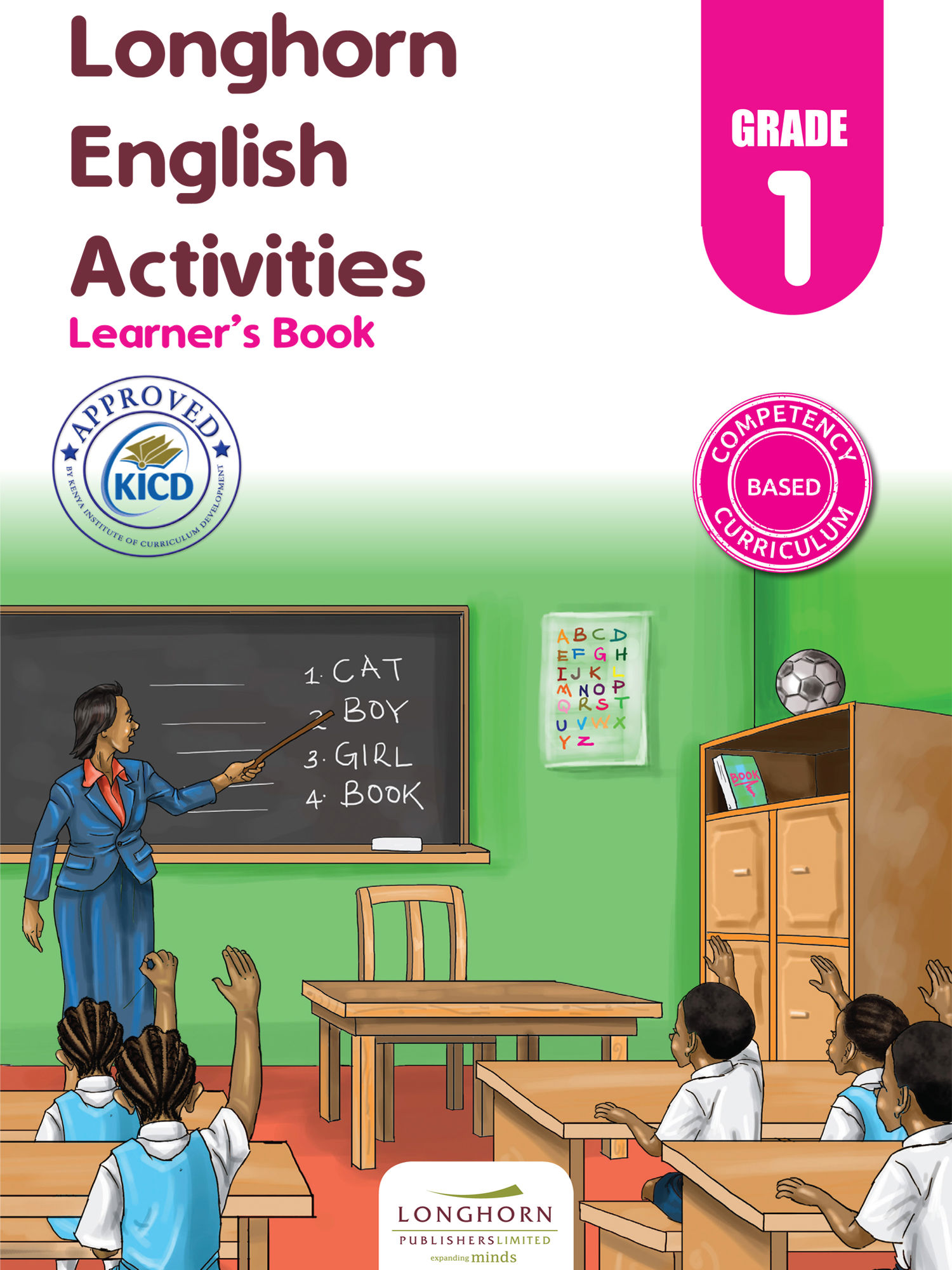Study kit (English 1)##### Authors
Tom Olang’ Okeyo, Naomi Kanyiri, Joseph Ayoro Ouma, Beatrice Mwebi, Immaculate Oundo
##### Exercises kit’s authors
Tom Olang’ Okeyo, Naomi Kanyiri, Joseph Ayoro Ouma, Beatrice Mwebi, Immaculate Oundo
##### Publisher
Longhorn Publishers
##### Included in packages• The study kit contains 90 chapters and teacher’s exercises kit contains 57 exercises.
• ##### Authors
Tom Olang’ Okeyo, Naomi Kanyiri, Joseph Ayoro Ouma, Beatrice Mwebi, Immaculate Oundo
• ##### Exercises kit’s authors
Tom Olang’ Okeyo, Naomi Kanyiri, Joseph Ayoro Ouma, Beatrice Mwebi, Immaculate Oundo
English
English
• ##### Publisher
Longhorn Publishers

1.1. Lesson 1
Free chapter!
1.2. Lesson 2
1.3. Lesson 3
1.4. Lesson 4
1.5. Lesson 5
1.6. Lesson 6

2.1. Lesson 1
2.2. Lesson 2
2.3. Lesson 3
2.4. Lesson 4
2.5. Lesson 5
2.6. Lesson 6

3.1. Lesson 1
3.2. Lesson 2
3.3. Lesson 3
3.4. Lesson 4
3.5. Lesson 5
3.6. Lesson 6

4.1. Lesson 1
4.2. Lesson 2
4.3. Lesson 3
4.4. Lesson 4
4.5. Lesson 5
4.6. Lesson 6

5.1. Lesson 1
5.2. Lesson 2
5.3. Lesson 3
5.4. Lesson 4
5.5. Lesson 5
5.6. Lesson 6

6.1. Lesson 1
6.2. Lesson 2
6.3. Lesson 3
6.4. Lesson 4
6.5. Lesson 5
6.6. Lesson 6

7.1. Lesson 1
7.2. Lesson 2
7.3. Lesson 3
7.4. Lesson 4
7.5. Lesson 5
7.6. Lesson 6

8.1. Lesson 1
8.2. Lesson 2
8.3. Lesson 3
8.4. Lesson 4
8.5. Lesson 5
8.6. Lesson 6

9.1. Lesson 1
9.2. Lesson 2
9.3. Lesson 3
9.4. Lesson 4
9.5. Lesson 5
9.6. Lesson 6

10.1. Lesson 1
10.2. Lesson 2
10.3. Lesson 3
10.4. Lesson 4
10.5. Lesson 5
10.6. Lesson 6

11.1. Lesson 1
11.2. Lesson 2
11.3. Lesson 3
11.4. Lesson 4
11.5. Lesson 5
11.6. Lesson 6

12.1. Lesson 1
12.2. Lesson 2
12.3. Lesson 3
12.4. Lesson 4
12.5. Lesson 5
12.6. Lesson 6

13.1. Lesson 1
13.2. Lesson 2
13.3. Lesson 3
13.4. Lesson 4
13.5. Lesson 5
13.6. Lesson 6

14.1. Lesson 1
14.2. Lesson 2
14.3. Lesson 3
14.4. Lesson 4
14.5. Lesson 5
14.6. Lesson 6